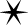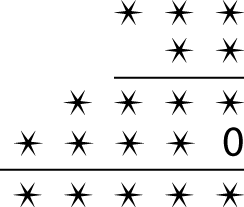#### You may also like### Repeaters

Choose any 3 digits and make a 6 digit number by repeating the 3 digits in the same order (e.g. 594594). Explain why whatever digits you choose the number will always be divisible by 7, 11 and 13.### Oh! Hidden Inside?

Find the number which has 8 divisors, such that the product of the divisors is 331776.### Skeleton

Amazing as it may seem the three fives remaining in the following `skeleton' are sufficient to reconstruct the entire long division sum.

# Long Multiplication

##### Age 11 to 14 Challenge Level:

A 3 digit number is multiplied by a 2 digit number and the calculation is written out as shown below.
Each star like this stands for one digit.Apart from the zero shown the only digits which occur are 2, 3, 5 and 7. This is sufficient information to complete the whole multiplication.This is sufficient information to complete the whole multiplication sum. You might find this sheet useful for working on the problem,